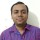Coffee Room
Discuss anything here - everything that you wish to discuss with fellow engineers.
12793 Members
Join this group to post and comment.C Question - 1

String S1 = "M, B , C , A , E "
String S2 = " X , Y , Z , A, C"

Problem:
Given String 1 and String 2, we need to write a program to find out the first occurance of character in string 2 which is present in string 1.

from example, in the above given string A is the character which is in s2 and s1 and which is found in S2 first.
got my question?
then write a C Program or give a procedure how you do it?
then I will come up with more question on this.Harshad Italiya • Mar 15, 2012
```unsigned char S1[]="MBCAE"

unsigned char S2[]="XYZAC"

unsigned char i,j,match;

void main()

{

for(i=0;i<5;i++)

{

for(j=0;j<5;j++)

{

if(S1==S2[j])

{

match =1;

break;

}

}

if(match==1)

break;

}

print(S1);

}

```
PS:- Haven't compile this code this is logic only It may be wrong.. 😉Ok, the logic is correct.

Here you are using two for loops, so the worst case scenario is you have the number of comparisions on the order of n[sup]2[sup].Now, I need ideas on how will you reduce the order of the program.

PS. THis is not my assignment or my project work 😛TO add curiosity , this is a Microsoft Interview question! faced by my friend.

Hink: Sorting technique will reduce the order to some extent.

There are other ways as well to reduce the order to only "n". So think and post your answers.simplycoder • Mar 18, 2012
Sort and use binary search. Where, sort can be implemented in O(n) time and binary search takes up O(lgn)Great! so we have reduced the order to nlogn now.

There is a way where we can reduce the way only to "n" ways.

That is using the hast table technique.Next question on the same one, what all scenarios you can think in testing the above code?

One is already done, we test the order and reduced it to N ways.

Consider string 1 and string 2 are pointers and give the test scenarios... There are many interesting things to learn. let me know your thoughts first.